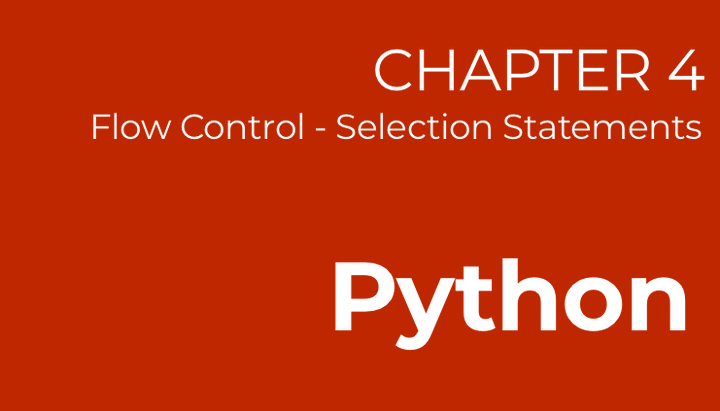# Python - Chapter 4

Flow Control (Selection Statements)Python programs run in sequence. This means that every line of code you type is executed as it is, in order from top to bottom, including the `import` statements, variable declarations, function declarations etc. During this sequence of execution, we can control the flow through two methods. The first is the use of selection statements which are also known as conditional statements.

## Using If, Elif and Else

Conditional statements in Python are declared using the `if`, `elif` and `else` keywords. Using this combination of keywords, we can check for various conditions which must be fulfilled before a subsequent block of code is executed.

``````a = 1
if(a == 1):
print("a is one")
else:
print("a is not one")``````

In the simple example above, we have used `if` and `else` to print out two different statements based on whether `a` is equivalent to 1 or not. The `==` operator simply checks for equality. The following table shows the full range of operators we can use.

OperatorMeaning
>Greater than
<Less than
!=Not equal to
>=Greater than or equal to
<=Less than or equal to

If we have more than 1 condition to check, we can use `elif` as shown below.

``````a = "apple"
if(a == "apple"):
print("Hello")
elif(a == "orange"):
print("Hi")
elif(a == "pear"):
print("Hola")
else:
print("Bye")``````

The above example uses the selection statements to execute three different branches, depending on the string we have assigned to `a`.

The exact program, if represented with a simple flowchart would look like this:

You will probably be using selection statements in every Python program you write. Let's give it a try now!

Exercise 3.1

Assign variable `a` a value of 500. Check if `a` is more 100, or more than 200 or more than 300. Print appropriate statements for each conditional check.

## Nested Statements

We can also nest the statements. For example, if we only had to use `if` and `else` keywords to do conditional checks, the following code will achieve just that.

``````if(marks == 90):
print("High Distinction")
else:
if(marks == 80):
print("Distinction")
else:
if(marks == 70):
print("Great")
else:
if(marks == 60):
print("Good")
else:
print("Try harder next time!")``````

As you see, what we get now is a deeply nested sequence of `if` and `else` statements. Though we will not do this since we have the `elif` statements, it shows that nesting these conditions is certainly possible!I code.